# Increasing and Decreasing Functions in Calculus

In calculus, derivative of a function used to check whether the function is decreasing or increasing on any intervals in given domain. For a given function, y = F(x), if the value of y is increasing on increasing the value of x, then the function is known as an increasing function and if the value of y is decreasing on increasing the value of x, then the function is known as a decreasing function.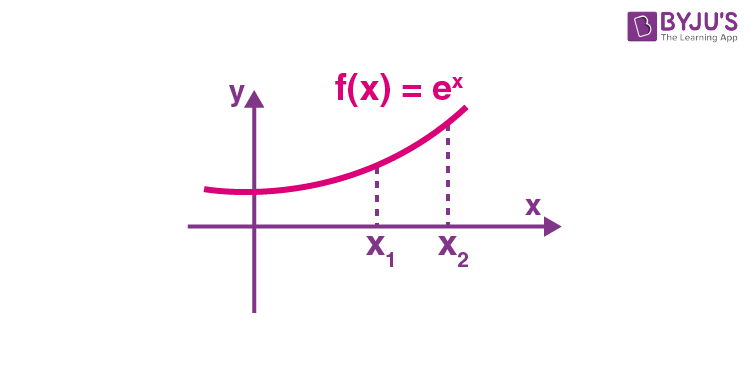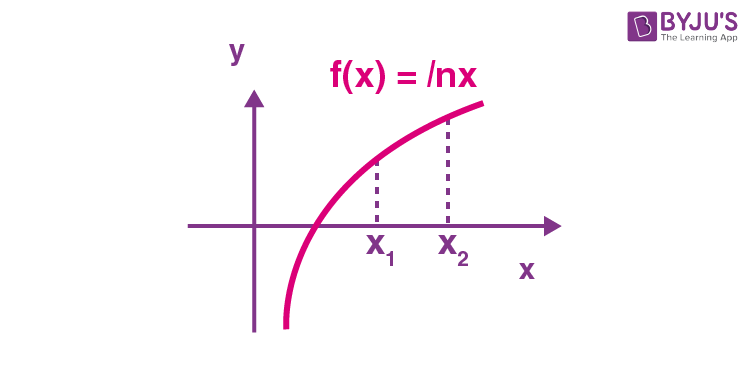In both of the given function x1 < x2 and F(x1) < F(x2), so we can say it is an increasing function.

F(x) = e-x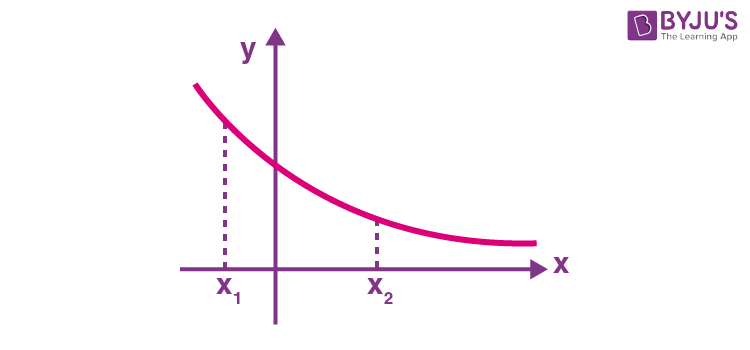In this function value of y is decreasing on increasing the value of x as x1 < x2 and F(x1) < F(x2)

## Increasing Function in Calculus

For a function, y = f(x) to be increasing $\frac{dy}{dx} \geq 0$ for all such values of interval (a, b) and equality may hold for discrete values.

Example: Check whether y = x3 is an increasing or decreasing function.

Solution: $\frac{dy}{dx} = 3x^2 \geq 0$

So, it is an increasing function.

Graphical Representation: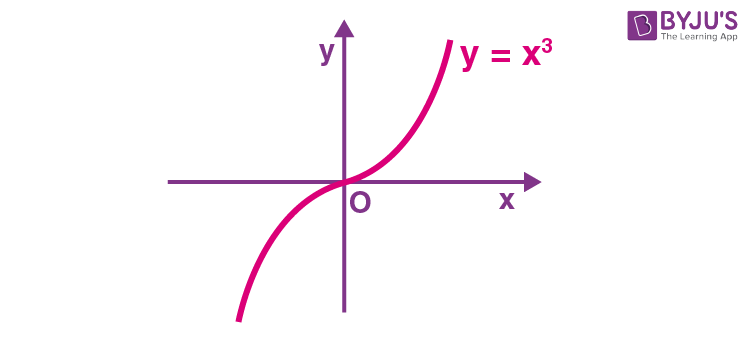## Decreasing Function in Calculus

For a function, y = f(x) to be monotonically decreasing $\frac{dy}{dx} \leq 0$

for all such values of interval (a, b) and equality may hold for discrete values.

Example: Check whether the function, y = -3x/4 + 7 is an increasing or decreasing function.

Differentiate the function with respect to x, we get

$\frac{dy}{dx} = -3/4 \leq 0$

So, we can say it is a decreasing function.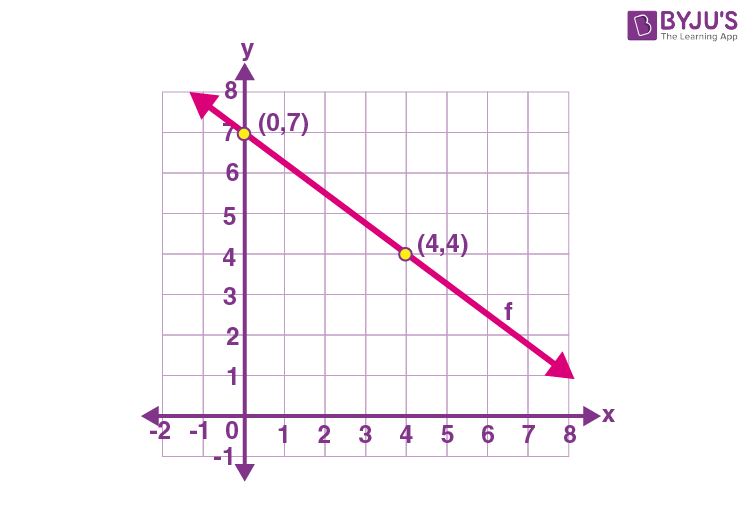## Solved Questions on Increasing and Decreasing Functions

Question 1: Prove that f(x) = x – sin(x) is an increasing function

Solution: f(x) = x – sin(x)

$\frac{dy}{dx}$ = 1 – cos(x)

$\frac{dy}{dx} \geq 0$ as cos(x) having value in interval [-1, 1] and $\frac{dy}{dx}$ = 0 for the discrete values of x and do not form an interval, hence we can include this function in monotonically increasing function.

Question 2: Prove that f(x) = cos x is decreasing function in [0, π]

Solution: f(x) = cos x

f’(x) = – sin x

As sin x is positive in first and second quadrant i.e. sin x ≥ 0 in [0, π], we can say that

$\frac{dy}{dx}$ = – (positive ) = negative ≤ 0

$\frac{dy}{dx} \leq 0$

So function f(x) = cos x is decreasing in [0, π].

Question 3: Prove that a polynomial with positive coefficients is increasing.

Solution: Let’s suppose polynomial is $a_1 x^n + a_2 x^{n-1} + a_3 x^{n-2}+…….+a_n x^1 + a_{n+1}x^0$

So, y = $a_1 x^n + a_2 x^{n-1} + a_3 x^{n-2}+…….+a_n x^1 + a_{n+1}x^0$

Derivate above function with respect to x, we have

$\frac{dy}{dx} = a_1 n x^{n-1} + a_2 (n-1)x^{n-2} + a_3 (n-2)x^{n-3}+…….+a_n + 0$

As every coefficient is positive and for a polynomial n ≥ 0

So $\frac{dy}{dx} \geq 0$

Therefore, we can say that a polynomial with positive coefficients is increasing.

Question 4: Discuss the increasing and decreasing nature of the function f(x) = x ln(x)

Solution:

Here, f(x) = x ln(x)

⇒ f’(x) = 1 + ln(x)

For a function to be increasing f’(x) > 0

⇒ 1 + ln(x) > 0

⇒ ln(x) > -1

⇒ ln(x) > – ln(e)

⇒ ln(x) > ln (e-1)

We know that ln(x) is increasing function, so for ln(x) > ln (e-1) to be hold

⇒ x > e-1

⇒ x > 1/e

Thus, function f(x) = x ln(x) to be increasing x ∈ (1/e, ∞) and for function f(x) = x ln(x) to be decreasing x ∈ (0, 1/e).

Question 5: Find the value of “a”, if the function x3 – 6x2 + ax to be increasing for all the value of x.

Solution:

Here f(x) = x3 – 6x2 + ax

⇒ f’(x) = 3x2 – 12x + a

For a function to be increasing f’(x) > 0

So, 3x2 – 12x + a > 0

⇒ 3(x2 – 4x + a/3) > 0

⇒ x2 – 4x + a/3 > 0

⇒ (x – 2)2 – 22 + a/3 > 0

⇒ (3(x – 2)2 – 12 + a)/3 > 0

⇒ 3(x – 2)2 – 12 + a > 0

We know that 3(x – 2)2 can’t be negative and having the minimum value 0 at x = 2

The minimum value of f’(x) is at x = 2

min. f’(x) = -12 + a

So for f’(x) > 0

⇒ -12 + a > 0

⇒ a > 12.